# Mercator projection

Introduction

Here on earth, we humans compare the sizes of different countries in terms of power. When the first digital map of the world came, people thought that some countries like Greenland are bigger than many other countries like Australia (As Greenland looked big on the map). Although it wasn't true. Some scientists actually created the model of sphere and found it wrong.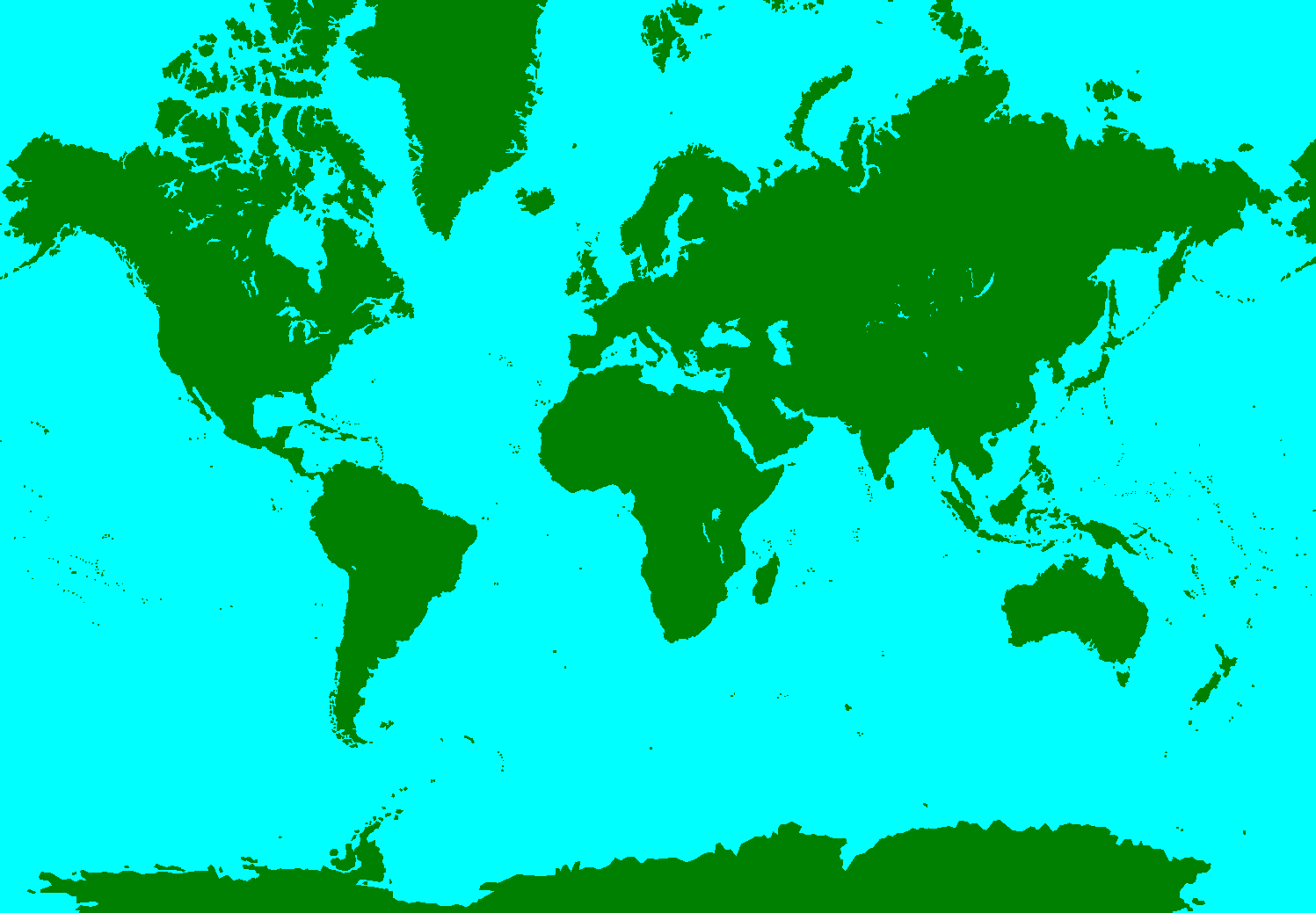Cylindrical Projections

The spherical approximation of Earth with radius a can be modelled by a smaller sphere of radius R, called the globe in this section. The globe determines the scale of the map. The various cylindrical projections specify how the geographic detail is transferred from the globe to a cylinder tangential to it at the equator. The cylinder is then unrolled to give the planar map.The fraction $\frac{R}{a}$ is called the representative fraction (RF) or the principal scale of the projection. For example, a Mercator map printed in a book might have an equatorial width of 13.4 cm corresponding to a globe radius of 2.13 cm and an RF of approximately $\frac{1}{300M}$ (M is used as an abbreviation for 1,000,000 in writing an RF) whereas Mercator's original 1569 map has a width of 198 cm corresponding to a globe radius of 31.5 cm and an RF of about $\frac{1}{20M}$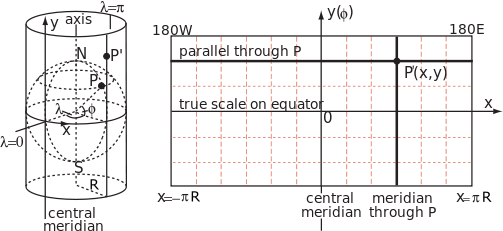.A cylindrical map projection is specified by formulae linking the geographic coordinates of latitude $\phi$ and longitude (\lamda ) to Cartesian coordinates on the map with origin on the equator and x-axis along the equator. By construction, all points on the same meridian lie on the same generator of the cylinder at a constant value of x, but the distance y along the generator (measured from the equator) is an arbitrary function of latitude, $y(\phi)$. In general this function does not describe the geometrical projection (as of light rays onto a screen) from the centre of the globe to the cylinder, which is only one of an unlimited number of ways to conceptually project a cylindrical map.

Since the cylinder is tangential to the globe at the equator, the scale factor between globe and cylinder is unity on the equator but nowhere else. In particular since the radius of a parallel, or circle of latitude, is $R\cos\phi$, the corresponding parallel on the map must have been stretched by a factor of $\frac{1}{\cos \phi }=\sec \phi$. This scale factor on the parallel is conventionally denoted by k and the corresponding scale factor on the meridian is denoted by h.

Actually comparing sizes

By comparing sizes of Greenland and Australia gives us: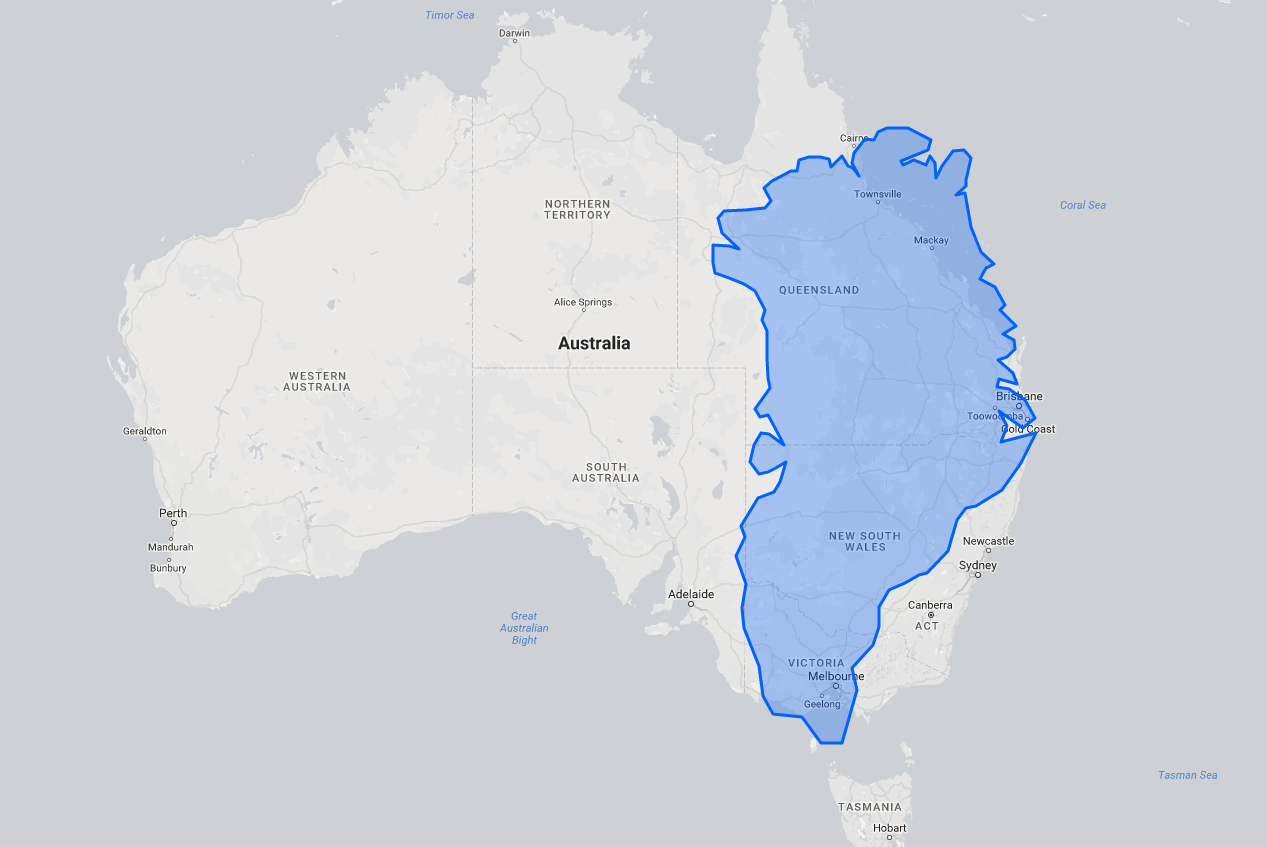Cleary, Australia is 3 times larger than Greenland. Again if we compare India and Greenland we get,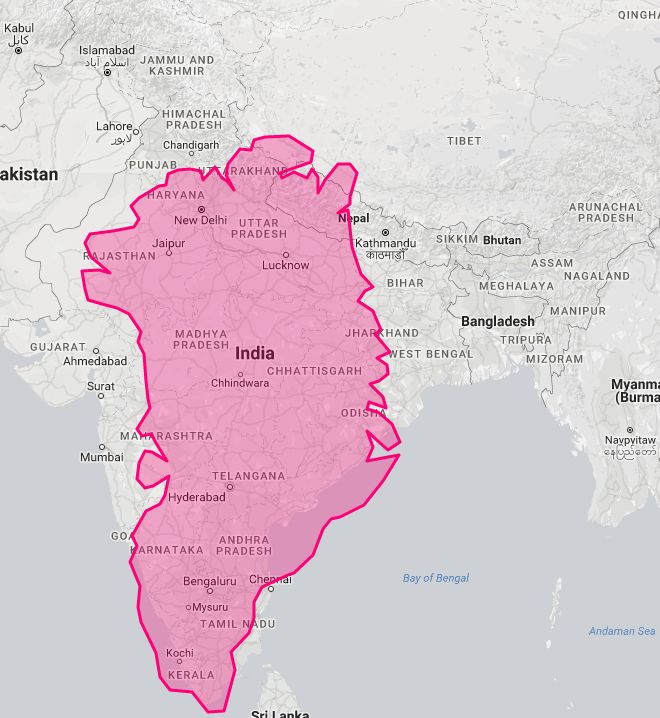Clearly, India is larger than Greenland.

If you want to actually compare the sizes of nations you can click this.

Hope you enjoyed reading this.

Source:Wikipedia,The True Size Of ....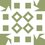Note by Sahil Silare
4 years, 5 months ago

This discussion board is a place to discuss our Daily Challenges and the math and science related to those challenges. Explanations are more than just a solution — they should explain the steps and thinking strategies that you used to obtain the solution. Comments should further the discussion of math and science.

When posting on Brilliant:

• Use the emojis to react to an explanation, whether you're congratulating a job well done , or just really confused .
• Ask specific questions about the challenge or the steps in somebody's explanation. Well-posed questions can add a lot to the discussion, but posting "I don't understand!" doesn't help anyone.
• Try to contribute something new to the discussion, whether it is an extension, generalization or other idea related to the challenge.
• Stay on topic — we're all here to learn more about math and science, not to hear about your favorite get-rich-quick scheme or current world events.

MarkdownAppears as
*italics* or _italics_ italics
**bold** or __bold__ bold
- bulleted- list
• bulleted
• list
1. numbered2. list
1. numbered
2. list
Note: you must add a full line of space before and after lists for them to show up correctly
paragraph 1paragraph 2

paragraph 1

paragraph 2

[example link](https://brilliant.org)example link
> This is a quote
This is a quote
    # I indented these lines
# 4 spaces, and now they show
# up as a code block.

print "hello world"
# I indented these lines
# 4 spaces, and now they show
# up as a code block.

print "hello world"
MathAppears as
Remember to wrap math in $$ ... $$ or $ ... $ to ensure proper formatting.
2 \times 3 $2 \times 3$
2^{34} $2^{34}$
a_{i-1} $a_{i-1}$
\frac{2}{3} $\frac{2}{3}$
\sqrt{2} $\sqrt{2}$
\sum_{i=1}^3 $\sum_{i=1}^3$
\sin \theta $\sin \theta$
\boxed{123} $\boxed{123}$

## Comments

There are no comments in this discussion.

×

Problem Loading...

Note Loading...

Set Loading...